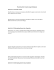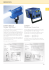# BS7671 Formula and Tips

## Transcription

BS7671 Formula and Tips
```BS7671 Formula and Tips
The earth fault loop impedance of that part of
the system external to the installation.
Ze = the external earth fault loop impedance of the
supply cable
Zs = the earth fault loop impendence of the Internal
current carrying circuit.
R1+R2 = the sum of the resistance of the line conductor
(R1) and the circuit protective conductor (R2) between
the point of utilisation and the origin of the installation.
Equitation: Zs = Ze + (R1 + R2)
Ohms Law
Resistance of the cable in ohms = R
Current being drawn from the circuit = I
Voltage being delivered to the circuit = V
Power being developed by the circuit = P
This can give us two triangles for formula
V
I
P
R
Resistance triangle
V
I
Power triangle
Ohms Law
V2
P
I
R
I2 X R
PxR
Power
P = watts
VxI
Power
P - watts
V
R
Current
I = amperes
Voltage
V = volts
IxR
Voltage
V - volts
V
I
Resistance
R = ohms
P
V
P
I2
P
R
V2
P
525 – Voltage drop in consumers’
installations
Regulation 525.3 directs readers to a table in
Appendix 12. pg 314
Description
Lighting
Other uses
(i) Low voltage installations supplied directly from a
public low voltage distribution system
3%
5%
(ii) Low voltage installation supplied from private LV
supply (*)
6%
8%
(*) The voltage drop within each final circuit should not exceed the values given in (i)
Where a distribution circuit (from origin) feeds another
distribution board, the total allowable voltage drop should be
split between the distribution circuit(s) and final circuit(s).
Voltage drop calculations
mV  I b  length
Voltage drop 
1000
Where the voltage drop is in volts and:
mV
Ib
Length
is the millivolts dropped per ampere per
metre taken from Tables 4D1A to 4J4A pg332-374
is the design current of the circuit (the
current intended to be carried)
is the length of the circuit in metres. The
division by 1000 gives the answer in volts.
Volt Drop
Cable Calculation
A lighting circuit protected by a BS3036 fuse consisting of 10 x 100W luminaires is wired in single copper pvc
700C insulated cable installed with two other circuits in conduit fixed to a wall.
The circuit length is 23m and the ambient temperature of the surroundings is 350C.
Carry out a cable calculation and check that the circuit complies with BS7671:2008 incorporating Amendment
no.1 2011.
Step 1. Gather the relevant information from the question.
•
10 x 100W luminaires = 1000W
700C PVC single copper cables run in conduit fixed to wall = Reference method B (BS7671 Table 4A2 p.317)
•
BS3036 protective device = Cf = 0.725 (BS7671 Appendix 4 p.308)
•
Circuit Length = 23m
•
Grouping with 2 other circuits (total 3) = Cg = 0.7 (BS7671 Table 4C1 p.327)
•
Ambient temperature 350C = Ca = 0.94 (BS7671 Table 4B1 p.325)
Volt Drop
Step 2. Calculate the circuit design current. (Ib) using ohms law power triangle I = P / V
Ib = P = 1000 =4.35A
V 230
Step 3. Determine the rating of the protective device (In)
In ≥ Ib = 5A (BS7671 Table 41.2 p.55)
Step 4. Determine the minimum current carrying capacity of the cable (It) (BS7671 Appendix 4 p.308)
It =
In
=
In
=
5
=
5 = 10.48A
Correction factors
(Cg x Ca x Cf )
(0.7 x 0.94 x 0.725)
0.477
Cable must be capable of carrying 10.48A
Volt Drop
Step 5. Identify a suitable cable. = (BS7671 Table 4D1A p.332 reference method B)
1mm2 cable has a rating of 13.5A
Step 6. Calculate the volt drop. (Vd) = (BS7671 Table 4D1B page 333 reference method A and B)
Voltage drop = (mV/A/m)x Ib x L = 44 x 4.35 x 23 = 4.4V
1000
1000
Appendix 4 page 314
This circuit complies with the requirements of BS7671 in that the calculated volt drop 4.4V is less than the
maximum permitted 3% for a single phase lighting circuits.
Single phase; 3% of 230 = 6.9V
Therefore the cable is suitable.
Resistance of copper and aluminium conductors
Uo = the normal voltage to earth
Zs = the earth fault loop impedance
and
: Zs = Ze + (R1 + R2)
Ze = the external earth fault loop impedance of the supply
cable
Zs = the earth fault loop impendence of the Internal current
carrying circuit.
R1+R2 = the sum of the resistance of the line conductor
(R1) and the circuit protective conductor (R2) between the
point of utilisation and the origin of the installation.
Resistance of copper and aluminium conduct
Similarly, in order to design circuits for compliance with BS7671
limiting values of earth fault loop impedance given in Tables 41.2
to 41.4, it is necessary to establish the relevant impedance of the
circuit conductors concerned at their operating temperatures.
For example 1.5mm2 with 1.0mm2 earth c.p.c. is given as
30.20 mΩ/m therefore if the cable is 20mts in length this will be
30.20 x 20 = 0.60Ω which is the value of R1+R2
1000
Electrical systems and earthing
arrangements
TN-S
TN-C-S
TT
TN-S system
Typical quoted
value for Ze is
0.8 W
TN-C-S system
Typical quoted
value for Ze is 0.35
W
TT system
This system
affords a
relatively high
Ze value, which
must not
exceed 21 W
Ra ≤ 200 W
TN-C system
• A TN-C system is uncommon in the United Kingdom.
• Neutral and protective functions are combined in a single
conductor (a PEN conductor) throughout the system
(although, the term CNE is sometimes used for such a
conductor forming part of the distributor’s lines).
• The exposed-conductive-parts of the installation are
connected to the PEN conductor, and hence to the earthed
point of the source of energy.
• Regulation 8(4) of the Electricity Safety, Quality and
Continuity Regulations 2002 prohibits the use of PEN
conductors in consumers’ installations.
Nominal Voltages in the UK
• Single phase (U0) 230 V (Actual 240 V)
• Three Phase (U) 400 V
(Actual 415 V)
IP code lettering/numbering systempg381
Discuss:
• IP2X,
• IP4X
• IPXXB, and
• IPXXD
‘LIVE’
TESTING
Prescribed tests:
Prescribed tests:
• Continuity
• Earth fault loop impedance
• Polarity
• Fault current measurement
• Insulation resistance
• RCD operation
Insure these items are tested
External Ze
Earth electrode RA (if applicable)
Continuity of protective conductors
Insulation between Live conductors and earth
Insulation between Live conductors
Polarity
Earth Loop-Impedance
Operation of Residual Current device
Functional Testing
VERIFICATION & CERTIFICATION - CERTIFICATES
Three types of Certificate could be used for a
domestic electrical installation:► An Periodic Inspection (Electrical Condition Report)
► An Electrical Installation Certificate
► A Minor
Works Certificate
Tips
Lets look at a typical question from the exam
and follow it through at various stages.
Example Question
BS7671 identifies that the cross-sectional area of a conductor shall be determined by
A
y
B
the nominal voltage
C
voltage tolerances
D
the earthing system
Tips
Now if we go straight to the BS7671 index and look up
cross-sectional area of conductors and cables page 442.
We find a reference to 132.6 Sec 524, we would now
look in part 1 chapter 32 regulation 6 page 19.
On reading the regulation we find:
The cross-sectional area of conductors shall be
determined for both normal operating conditions and
where appropriate, for fault conditions according to:
( 1 ) the admissible maximum temperature.
Therefore we know that ( A ) must be the answer.
Example Question
BS7671 identifies that the cross-sectional area of a conductor shall be determined by
A
B
the nominal voltage
C
voltage tolerances
D
the earthing system
Tips
Lets try another example and follow that through!
Example Question
The algebraic sum of the currents in the live conductors of a circuit at a point in the electrical installation
is known as the
A
y
residual current
B
harmonic current
C
line current
D
neutral current
Tips
Looking in the BS7671 index the only phrase we can
find from the question is Electrical installation
definition Part 2 page 444.
We then go to Part 2 Definitions we can only find
Residual current. Algebraic sum of the currents in the
live conductors of a circuit at a point in the electrical
installation. Page 33
We now know that ( A ) is the answer.
Example Question
The algebraic sum of the currents in the live conductors of a circuit at a point in the electrical installation
is known as the
A
residual current
B
harmonic current
C
line current
D
neutral current
Tips
As we can see from the last question it is not
always plain sailing in finding out an answer!
But keeping calm and looking in the question for
the various words or the answers, you can find
what you are looking for. Now you try the next
one!
Example Question
Which one of the following cannot be used as basic protection
A
y
insulation of live parts
B
barriers or enclosures
C
protective earthing and bonding
D
obstacles
Which one of the following cannot be used as basic protection
Options a,b,d, all provide basic protection (see Section 416 and 417) option c provides fault protection
A
y
insulation of live parts
B
barriers or enclosures
C
protective earthing and bonding
D
obstacles
```

### The Z-Brick® is a peice of test equipment to quickly check the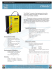### ie rules for safety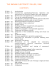### KIRCHOFF`S VOLTAGE LAW: EXAMPLE 1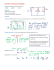### Sample ELEC 311 Final Questions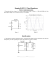### INF5481 RF Circuit, Theory and Design Assignment #3 Problem 1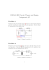### From the Desk of Dean G. De Vries Bock - ELCSA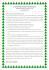### Vincent Camus